# isocubes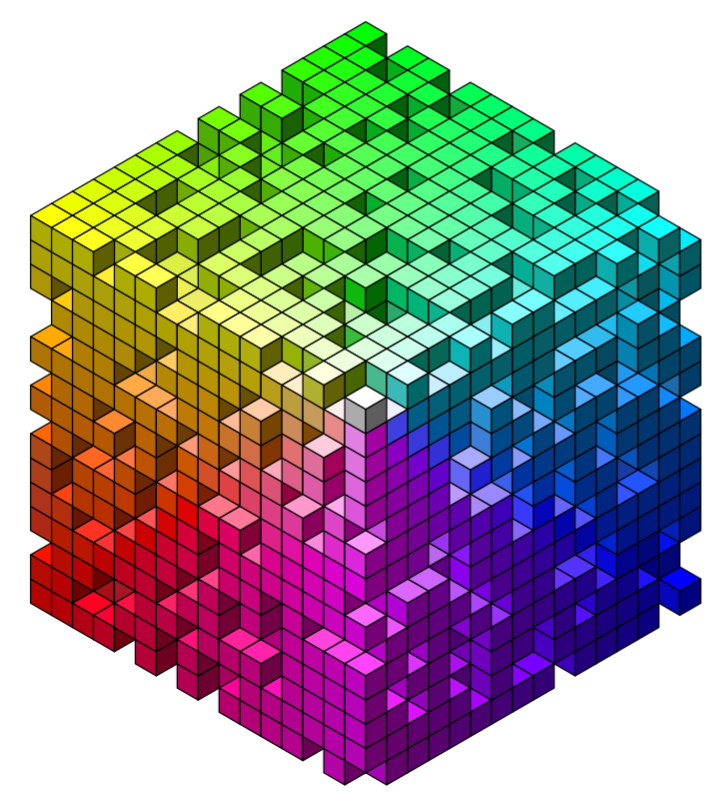The purpose of this package is to provide a 3D rendering backend for a very particular visual aesthetic.

That is, {isocubes} is an isometric rendering canvas with cubes as the only graphics primitive.

Some tools are included for creating particular scenes, but in general, if you can provide a list of (x,y,z) integer coorindates of what to render, then isocumes will create a 3d render.

## What’s new in v0.1.2

• Signed distance fields for creating objects

## Installation

You can install from GitHub with:

# install.package('remotes')
remotes::install_github('coolbutuseless/isocubes')

## Fake Terrain with ambient

library(grid)
library(ggplot2)
library(dplyr)
library(ambient)

#~~~~~~~~~~~~~~~~~~~~~~~~~~~~~~~~~~~~~~~~~~~~~~~~~~~~~~~~~~~~~~~~~~~~~~~~~~~~
# Create some perline noise on an NxN grid
#~~~~~~~~~~~~~~~~~~~~~~~~~~~~~~~~~~~~~~~~~~~~~~~~~~~~~~~~~~~~~~~~~~~~~~~~~~~~
set.seed(3)
N <- 60

dat <- long_grid(x = seq(0, 10, length.out = N), y = seq(0, 10, length.out = N)) %>%
mutate(
noise =
gen_perlin(x, y, frequency = 0.3) +
gen_perlin(x, y, frequency = 2) / 10
)

hm <- dat %>%
mutate(
x = x * 4,
z = y * 4,
y = noise * 4
)

pal  <- topo.colors(11)
sy   <- as.integer(10 * (hm$y - min(hm$y)) / diff(range(hm$y))) + 1 cols <- pal[sy] cubes <- isocubesGrob(hm, ysize = 1/45, xo = 0.7, fill = cols, col = NA) grid.newpage(); grid.draw(cubes)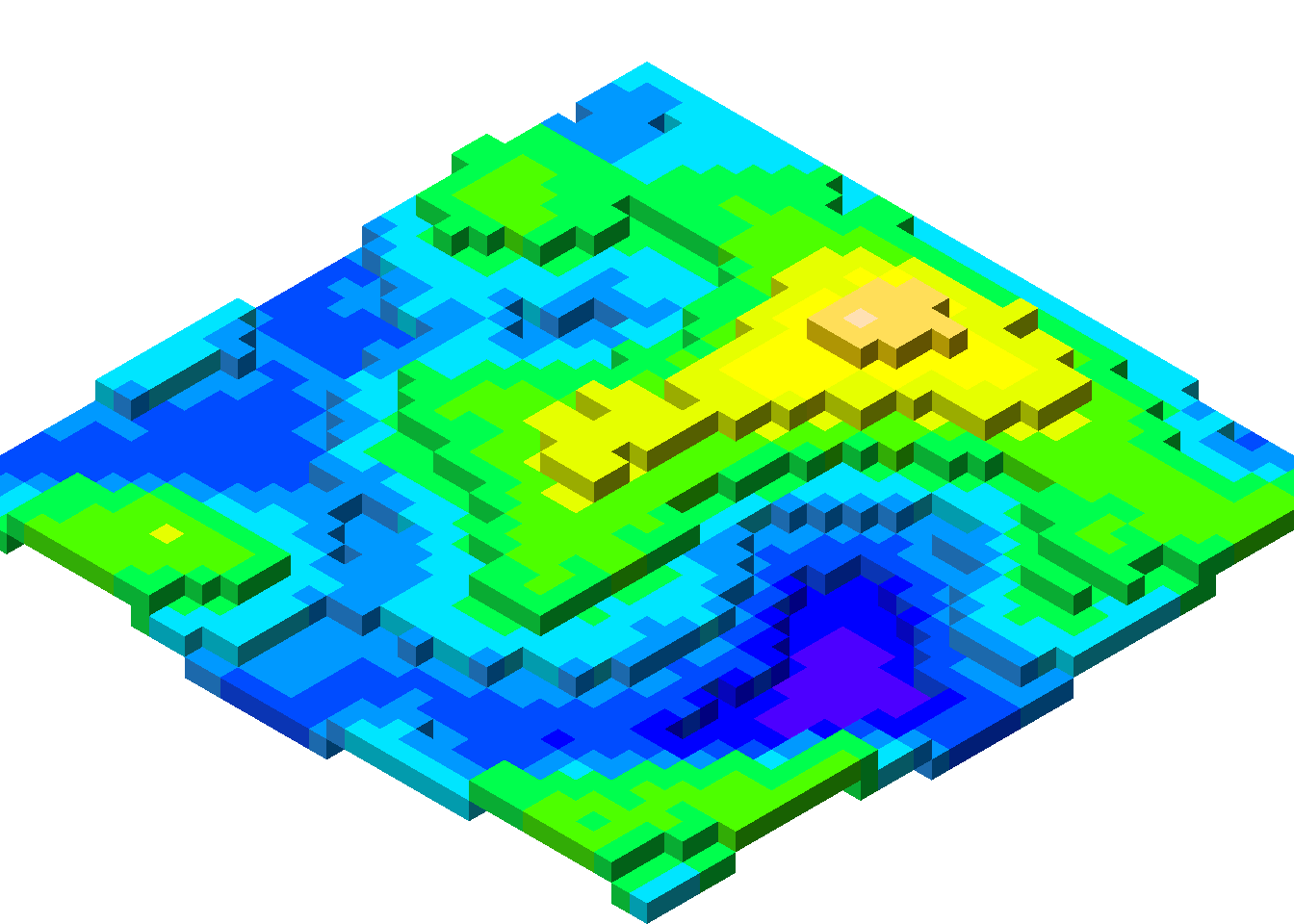## Bitmap font rendering library(grid) library(isocubes) library(bdftools) bdf <- bdftools::read_bdf_builtin("spleen-32x64.bdf") single_word <- bdftools::bdf_create_df(bdf, "#RStats!") N <- 10 cols <- rainbow(N) multiple_words <- purrr::map_dfr(seq(N), function(i) { single_word$z <- i
single_word$col <- cols[i] single_word }) cubes <- isocubesGrob(multiple_words, ysize = 1/170, xo = 0.1, fill = multiple_words$col)
grid.newpage(); grid.draw(cubes)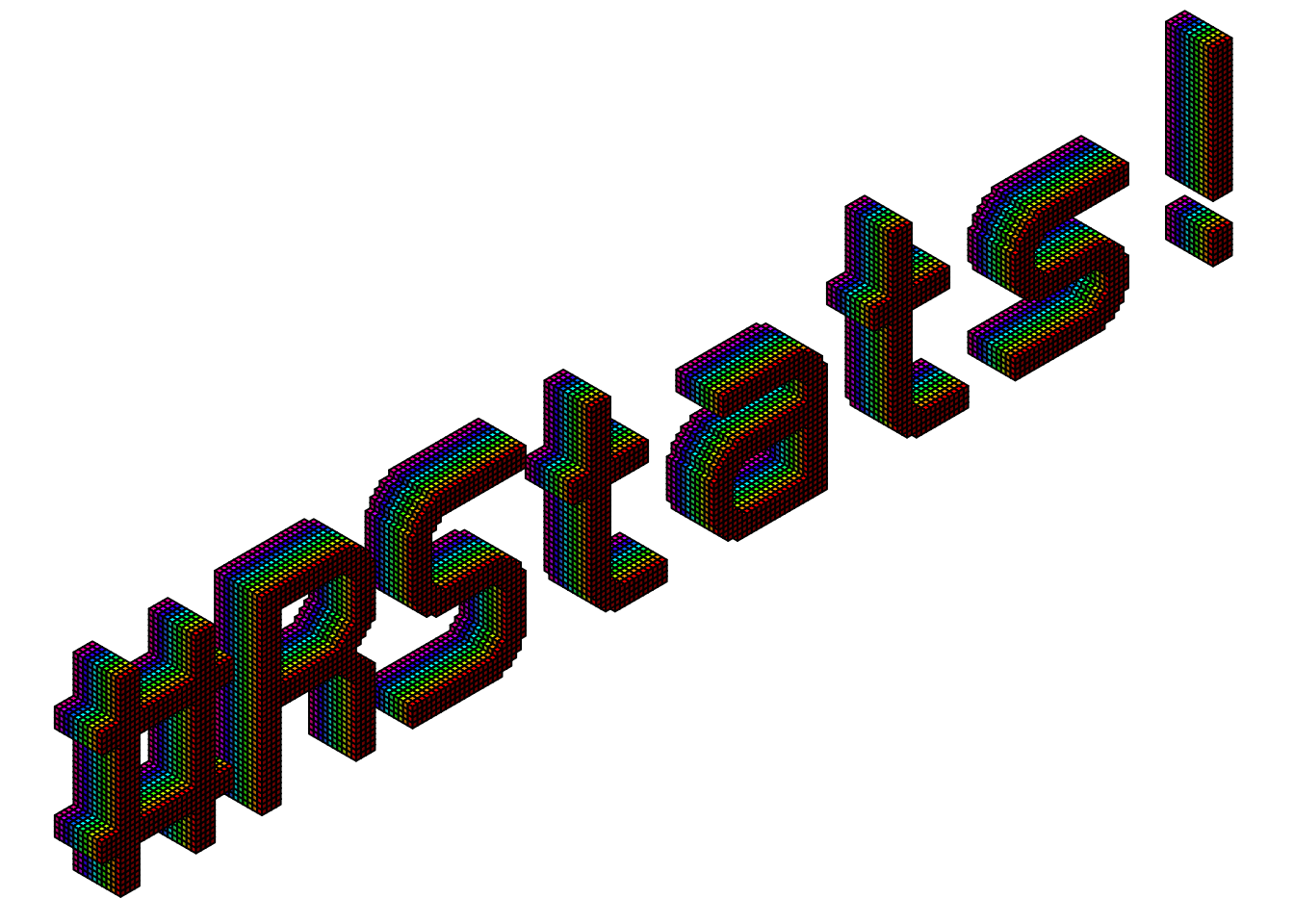## Signed Distance Fields - Simple

library(grid)
library(dplyr)
library(isocubes)

# Create a scene that consists of a scaled torus
scene <- sdf_torus(3, 1) %>%
sdf_scale(5)

# Render the scene into a list of coordinates of voxels inside objects
coords <- sdf_render(scene, N = 30)

# Create cubes, and draw
cubes  <- isocubesGrob(coords, ysize = 1/50, xo = 0.5, yo = 0.5, fill = 'lightblue')
grid.newpage(); grid.draw(cubes)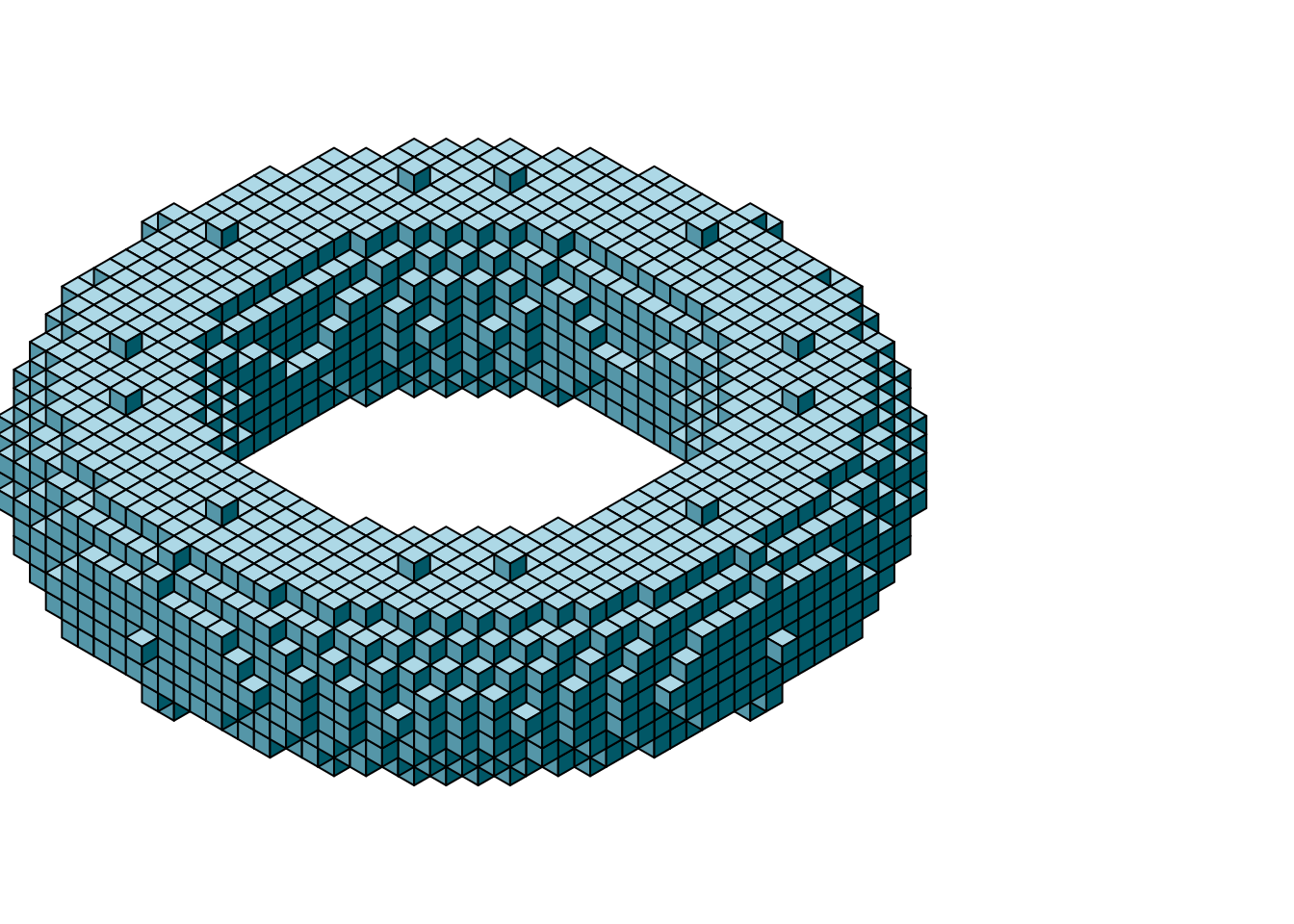## Signed Distance Fields - More Complex

library(dplyr)
library(grid)
library(isocubes)

sphere <- sdf_sphere() %>%
sdf_scale(40)

box <- sdf_box() %>%
sdf_scale(32)

cyl <- sdf_cyl() %>%
sdf_scale(16)

scene <- sdf_subtract_smooth(
sdf_intersect(box, sphere),
sdf_union(
cyl,
sdf_rotatey(cyl, pi/2),
sdf_rotatex(cyl, pi/2)
)
)

coords <- sdf_render(scene, 50)
cubes  <- isocubesGrob(coords, ysize = 1/100, xo = 0.5, yo = 0.5, fill = 'lightseagreen')
grid.newpage(); grid.draw(cubes)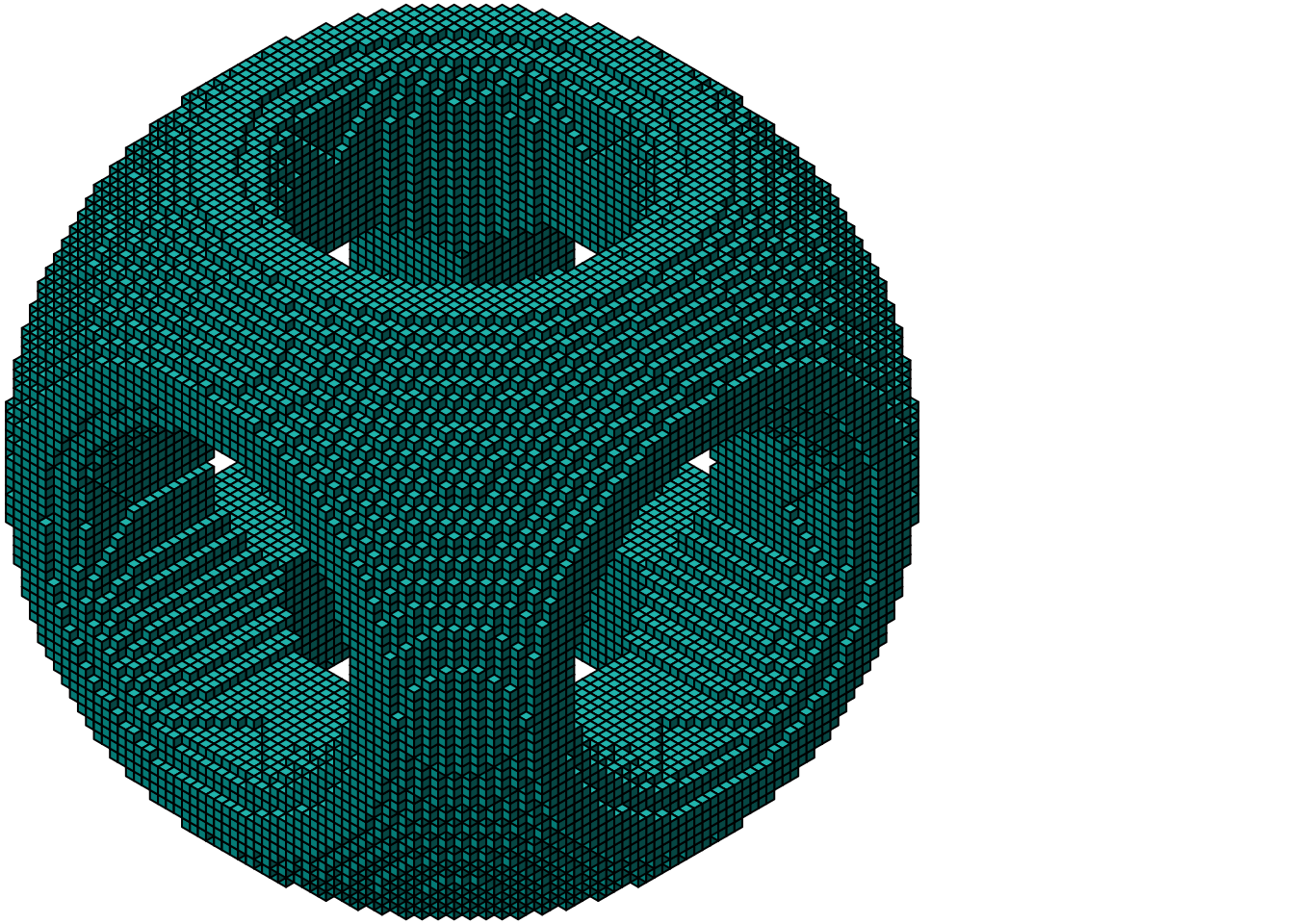## Signed Distance Fields - Animated

Unfortunately this isn’t fast enough to animate in realtime, so I’ve stitched together individuall saved frames to create an animation.

thetas <- seq(0, pi, length.out = 45)

for (i in seq_along(thetas)) {
cat('.')
theta <- thetas[i]

rot_scene <- scene %>%
sdf_rotatey(theta) %>%
sdf_rotatex(theta * 2) %>%
sdf_rotatez(theta / 2)

coords <- sdf_render(rot_scene, 50)
cubes  <- isocubesGrob(coords, ysize = 1/110, xo = 0.5, yo = 0.5)

png_filename <- sprintf("working/anim/%03i.png", i)
png(png_filename, width = 800, height = 800)
grid.draw(cubes)
dev.off()
}

# ffmpeg -y -framerate 20 -pattern_type glob -i 'anim/*.png' -c:v libx264 -pix_fmt yuv420p -s 800x800 'anim.mp4'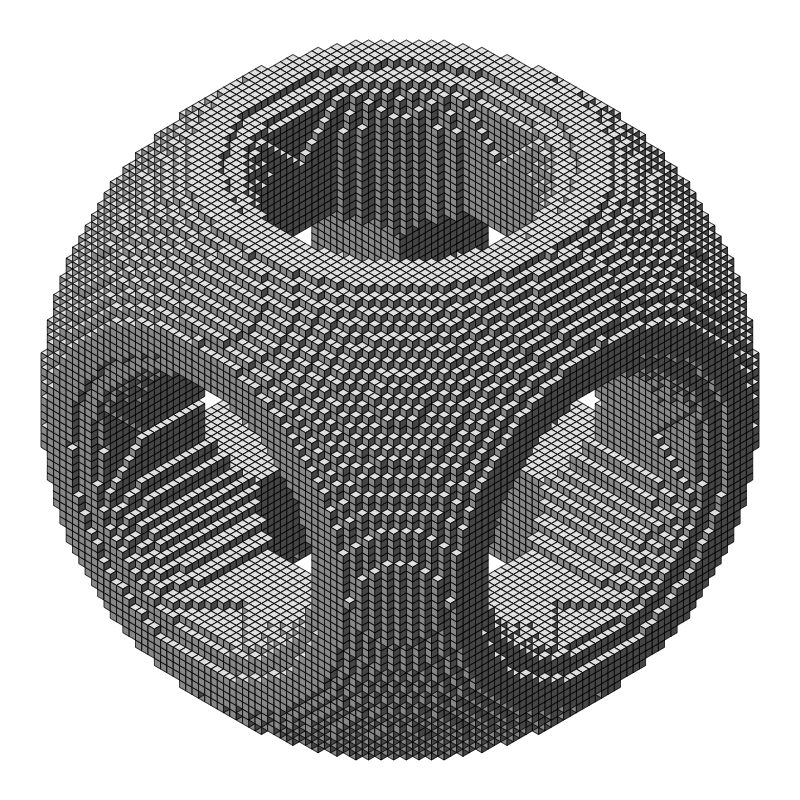## Technical Bits

#### Cube occlusion

In an isometric view, a cube at position (x, y, z) will block the view of any cube at (x + n, y - n, z + n).

Since cube positions must be integer values, hashes of cube positions are calculated as x + (y * 256) + (z * 256^2).

For each initial cube position, calculate the hash or coordinates of several occluded cubes. Then remove any initial cubes which match the occluded cube hashes.

#### Cube sort

Arrange cubes by -x, -z then y to ensure cubes are drawn in the correct ordering such that cubes in front are drawn over the top of cubes which are behind.

#### grob

All the faces of all the cubes are then calculated as polygons - each with 4 vertices.

The data for all polygons is then concatenated into a single polygonGrob() call with an appropiate vector for id.lengths to split the data.

#### Prototyping

Most of the prototyping for this package was done with {ingrid} - a package I wrote which I find makes working iteratively/at-the-console with base grid graphics a bit easier.

## Acknowledgements

• R Core for developing and maintaining the language.
• CRAN maintainers, for patiently shepherding packages onto CRAN and maintaining the repository